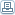Undervalued Stocks : Valuing Stocks through Intrinsic Value

 By: John B KeownThe formula as described by Graham, is as follows:Value = Current (Normal) Earnings x (8.5 + (2 x Expected Annual Growth Rate)Where the Expected Annual Growth Rate "should be that expected overthe next seven to ten years."The value of 8.5 appears to be the P/E ratio of a stock that has zerogrowth. It is not clear from the text how Graham arrived at this figure, but it is likely it represents the y-intercept of a normal distribution of a series of various P/E values plotted against corresponding growth figures.Graham's formula takes no account of prevailing interest rates; at the timehe last updated the chapter, around 1971, the yield on AAA Corporate Bondswas around 4.4%. We can adjust the formula by normalizing it for currentbond yields by multiplying by a factor of 4.40/{Current AAA Corp Bond Yield}. Bond yields can be found on Yahoo!Lets take a real-life example, using IBM. According to Yahoo!, the expectedgrowth rate for IBM over the next 5 years is 10% per annum (note data isonly available for 5 years ahead rather than the 7-10 years Graham states, but this should not make a significant difference). EPS for IBM over the last 12 months is \$4.95. Taking these values and plugging in the 20 year AA Corporate bond yield of 5.76% (AA Bond yields are higher than AAA so will give a more conservative estimate of IV) in our adjustment gives:Intrinsic Value = 4.95 x (8.5 + (2 x 10) x (4.40/5.76) = \$107.77IBM is currently trading at around \$91, so it is currently slightly undervalued.We can also do the same calculation for IBM's average expected 2005 earnings of \$5.62 in order to give some idea of what IBM's price shouldbe if it meets those earnings estimates:Intrinsic Value = 5.62 x (8.5 + (2 x 10) x (4.40/5.76) = \$122.36Of course this calculation is somewhat subjective when considered on its own. It should never be used in isolation - we must always take intoaccount other factors such as debt/equity, cash flow, managementeffectiveness, prevailing economic conditions, etc. Investors should seeksome qualifying criteria such as a PEG (Price Earnings Growth) ratio of less than 1 in additon to the stock being undervalued based on trailing and forward intrinsic value. Be aware that PEG itself is also based on future expectations, so we have to have some degree of certainty that thecompany will meet those expectations. We can do this by looking at thelast 5 years growth rate and Earnings figures.There are, of course, other methods of calculating intrinsic value butthis is certainly one of the simplest.(c) 2005 You may use this article, as-is, provided this copyright notice is kept intact.

Most Read Articles On